# The SEQDESIGN Procedure

### PROC SEQDESIGN Statement

Subsections:
• PROC SEQDESIGN <options>;

The PROC SEQDESIGN statement invokes the SEQDESIGN procedure. Table 101.1 summarizes the options available in the PROC SEQDESIGN statement.

Table 101.1: Summary of PROC SEQDESIGN Options

Option

Description

Design Parameters

Specifies the alternative reference

Specifies the statistic scale for the boundary

Specifies the maximum information level

Table Output

Displays the cumulative error spending at each stage

Displays powers and expected sample sizes

Displays expected cumulative stopping probabilities

Graphics Output

Displays the expected sample numbers plot

Displays the detailed boundary plot

Displays the combined boundary plot

Displays the error spending plot

Displays the powers plot

By default, the SEQDESIGN procedure displays tables of design information, method information, and boundary information for each specified design. If ODS Graphics is enabled, it also displays a detailed boundary plot.

In addition, you can use output options to display output tables such as expected cumulative stopping probability at each stage under various hypothetical references. If ODS Graphics is enabled, you can also use output options to display plots such as powers and expected sample sizes under various hypothetical references.

The following options can be used in the PROC SEQDESIGN statement to derive boundary values for all sequential designs in the procedure. They are listed in alphabetical order.

ALTREF=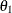<( <LOWER=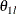>   <UPPER=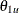> )>

specifies the alternative reference—that is, the hypothetical reference under the alternative hypothesis at which the power is computed. The LOWER= and UPPER= options are applicable only for a two-sided design with different lower and upper alternative references.

For a one-sided design,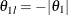is the lower alternative reference and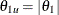is the upper alternative reference. For a two-sided design, the specifiedandare the lower and upper alternative references, respectively. If the LOWER= option is not specified,, and if the UPPER= option is not specified,.

The specification of the ALTREF= option depends on the hypothesis used in the clinical trial. For example, suppose the null hypothesis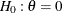with an alternative hypothesis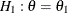is used to compare two binomial populations,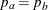. Thenis the proportion difference under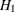if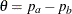, andis the log odds ratio underif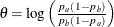.

If the ALTREF= option is not specified, the alternative referencecan also be specified or derived in the SAMPLESIZE statement. Ifis specified or derived in the SAMPLESIZE statement,andare the lower and upper alternative references, respectively.

Note that if the SAMPLESIZE statement is specified with a two-sided design, the sample sizes derived by using the lower and upper alternatives might be different. Ifis specified or derived in the SAMPLESIZE statement, it is used to compute the sample sizes. Otherwise, thespecified in the ALTREF= option is used.

BOUNDARYSCALE=MLE | SCORE | STDZ | PVALUE
BSCALE=MLE | SCORE | STDZ | PVALUE

specifies the scale for the statistic that is displayed in the boundary table and boundary plots. The keywords MLE, SCORE, STDZ, and PVALUE correspond to the boundary with the maximum likelihood estimate scale, the score statistic scale, the standardized normal Z scale, and the p-value scale, respectively. The default is BOUNDARYSCALE=STDZ.

With the BOUNDARYSCALE=MLE or BOUNDARYSCALE=SCORE option, the maximum information must be either explicitly specified with the MAXINFO= option or derived in the SEQDESIGN procedure to provide the necessary information level at each stage to compute the boundary values. See the section Boundary Scales for a detailed description of the statistic scale for the boundary values.

Note that for a two-sided design, the p-value scale displays the one-sided fixed-sample p-value under the null hypothesis with a lower alternative hypothesis.

MAXINFO=number

specifies the maximum information level for the design. If the MAXINFO=option is specified and the alternative reference is either specified explicitly with the ALTREF= option or derived from the SAMPLESIZE statement, then the Type I and Type II error probability levels cannot be met simultaneously. In this case, the ALPHA= option in the DESIGN statement is applicable only with the BOUNDARYKEY=ALPHA option (which is the default) in the DESIGN statement, and the Type II error probability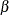is derived. The BETA= option in the DESIGN statement is applicable only with the BOUNDARYKEY=BETA option in the DESIGN statement, and the Type I error probabilityis derived.

#### Table Output Options

The following options can be used in the PROC SEQDESIGN statement to display addition table output. They are listed in alphabetical order.

ERRSPEND

displays the error spending at each stage for each boundary in the design.

PSS <( CREF=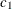<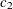…> )>

displays powers and expected sample sizes under various hypothetical references, where the numbers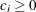.

For a one-sided design, the power and expected sample sizes under hypotheses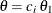are displayed, whereis the alternative reference and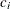are the values specified in the CREF= option.

For a two-sided design, the power and expected sample sizes under hypotheses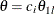and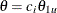are displayed, whereandare the lower and upper alternative references, respectively. The default is CREF= 0 0.5 1.0 1.5.

Note that for a symmetric two-sided design, only the power and expected sample sizes under hypotheses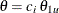are derived. See the section Type I and Type II Errors for a detailed description of the power computation. See the section Powers and Expected Sample Sizes for a detailed description of the expected sample size computation.

STOPPROB <( CREF=<…> )>

displays expected cumulative stopping probabilities under various hypothetical references, where the numbers.

For a one-sided design, expected cumulative stopping probabilities at each stage under hypothesesare displayed, whereis the alternative reference andare the values specified in the CREF= option.

For a two-sided design, expected cumulative stopping probabilities at each stage under hypothesesandare displayed, whereandare the lower and upper alternative references, respectively. Note that for a symmetric two-sided design, only expected cumulative stopping probabilities under hypothesesare derived. The default is CREF= 0 0.5 1.0 1.5.

#### Graphics Output Options

This section describes the options for using ODS Graphics with the SEQDESIGN procedure to create plots.

ODS Graphics must be enabled before plots can be requested. For example:

ods graphics on;
proc seqdesign altref=-10 plots=boundary(hscale=samplesize);
TwoSidedOBrienFleming: design nstages=4 method=obf;
samplesize model=twosamplemean(stddev=20);
run;


For more information about enabling and disabling ODS Graphics, see the section Enabling and Disabling ODS Graphics in Chapter 21: Statistical Graphics Using ODS.

The following options can be used in the PROC SEQDESIGN statement to display graphs with ODS Graphics. They are listed in alphabetical order.

PLOTS <( ONLY )> <= plot-request>
PLOTS <( ONLY )> <= ( plot-request < …plot-request> ) >

specifies options that control the details of the plots. The default is PLOTS=BOUNDARY. The global plot option ONLY suppresses the default plots and displays only plots specifically requested.

The plot request options are as follows.

ALL

produces all appropriate plots.

ASN <( CREF=<…> )>

displays a plot of the average sample numbers (expected sample sizes for nonsurvival data or expected numbers of events for survival data) under various hypothetical references, where the numbers. These average sample numbers are displayed as percentages of the average sample numbers for the corresponding fixed-sample design.

For a one-sided design, expected sample numbers under hypothesesare displayed, whereis the alternative reference andare the values specified in the CREF= option.

For a two-sided design, expected sample numbers under hypothesesandare displayed, whereandare the lower and upper alternative references, respectively. Note that for a symmetric two-sided design, only the average sample numbers under hypothesesare derived. The default is CREF= 0 to 1.5 by 0.01.

BOUNDARY <( HSCALE=INFO | SAMPLESIZE  ) >

displays a plot of the resulting sequential boundaries with the acceptance and rejection regions for each design. Either the information level (HSCALE=INFO) or the sample size (HSCALE=SAMPLESIZE) is displayed on the horizontal axis. If the maximum information is not available for the design, the information in percentage of its corresponding fixed-sample design are used in the plot. The stage number for each stage is displayed inside the plot. The default is HSCALE=INFO.

If the HSCALE=SAMPLESIZE option is specified. the SAMPLESIZE statement must also be specified. The options MODEL=INPUTNEVENTS, MODEL=TWOSAMPLESURVIVAL, and MODEL=PHREG in the SAMPLESIZE statement indicate survival data. For a sample that does not contain survival data, the sample size at each stage is displayed on the horizontal axis. For survival data, the number of events is displayed on the horizontal axis at each stage. The critical values for the corresponding fixed-sample design are also displayed in the plot.

COMBINEDBOUNDARY <( HSCALE=INFO | SAMPLESIZE | STAGE ) >

displays a plot of the resulting sequential boundaries for all designs simultaneously. You can display the information level (HSCALE=INFO), the sample size (HSCALE=SAMPLESIZE), or the stage number (HSCALE=STAGE) on the horizontal axis. The default is HSCALE=INFO. With HSCALE=INFO, if the maximum information is not available for the design, then the information in percentage of its corresponding fixed-sample design is used in the plot.

If the HSCALE=SAMPLESIZE option is specified, the SAMPLESIZE statement must also be specified. The options MODEL=INPUTNEVENTS, MODEL=TWOSAMPLESURVIVAL, and MODEL=PHREG in the SAMPLESIZE statement indicate survival data. For a sample that does not contain survival data, the sample size at each stage is displayed on the horizontal axis. For survival data, the number of events is displayed on the horizontal axis at each stage.

ERRSPEND <( HSCALE=INFO | STAGE ) >

displays a plot of the error spending for all sequential boundaries in the designs simultaneously. You can display the information level (HSCALE=INFO) or the stage number (HSCALE=STAGE) on the horizontal axis. With HSCALE=INFO, the information fractions are used in the plot. The default is HSCALE=STAGE.

NONE

suppresses all plots.

POWER <( CREF=<…> ) >

displays a plot of the power curves under various hypothetical references, where the numbers.

For a one-sided design, powers under hypothesesare displayed, whereis the alternative reference andare the values specified in the CREF= option.

For a two-sided design, powers under hypothesesandare displayed, whereandare the lower and upper alternative references, respectively. Note that for a symmetric two-sided design, only powers under hypothesesare derived. The default is CREF= 0 to 1.5 by 0.01.